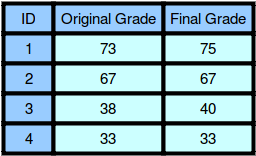আগামী ৩০ সেপ্টেম্বর থেকে Professional web Design and Development with HTML, CSS, JAVASCRIPT, PHP, MySQL, JQUERY and AJAX কোর্স এর ৯৪ তম ব্যাচ এবং ২৭ সেপ্টেম্বর থেকে Zend (PHP 7.1) Certified PHP Engineering (ZCPE) কোর্স এর ৪৪ তম ব্যাচ শুরু হতে যাচ্ছে। সীমিত সংখ্যক আসন বাকি আছে। আগ্রহীদেরকে অতিসত্বর মাসুদ আলম স্যার এর সাথে যোগাযোগ করার জন্য অনুরোধ করা যাচ্ছে। স্যার এর মোবাইল নম্বর : ০১৭২২ ৮১ ৭৫ ৯১

HackerLand University has the following grading policy:

• Every student receives a in the inclusive range from to .
• Any less than is a failing grade.

Sam is a professor at the university and likes to round each student’s according to these rules:

• If the difference between the and the next multiple of is less than , round up to the next multiple of .
• If the value of is less than , no rounding occurs as the result will still be a failing grade.

For example, will be rounded to but will not be rounded because the rounding would result in a number that is less than .

Given the initial value of for each of Sam’s students, write code to automate the rounding process. For each , round it according to the rules above and print the result on a new line.

Input Format

The first line contains a single integer denoting (the number of students).
Each line of the subsequent lines contains a single integer, , denoting student ‘s grade.

Constraints

Output Format

For each of the grades, print the rounded grade on a new line.

Sample Input 0

4
73
67
38
33


Sample Output 0

75
67
40
33


Explanation 01. Student received a , and the next multiple of from is . Since , the student’s grade is rounded to .
2. Student received a , and the next multiple of from is . Since , the grade will not be modified and the student’s final grade is .
3. Student received a , and the next multiple of from is . Since , the student’s grade will be rounded to .


<?php

$handle = fopen ("php://stdin","r"); fscanf($handle,"%d",$n); for($a0 = 0; $a0 <$n; $a0++){ fscanf($handle,"%d",$grade);$next = round($grade/5) * 5;$condition = $next -$grade < 3;

if ( $next >$grade && is_float($grade / 5) &&$condition && $grade > 37) { if (!$condition) {
echo $grade."\n"; }else{ echo$next."\n";
}
}else{

1.Maryam malik